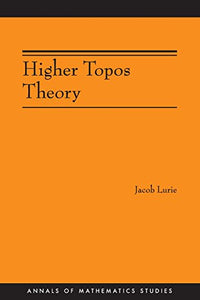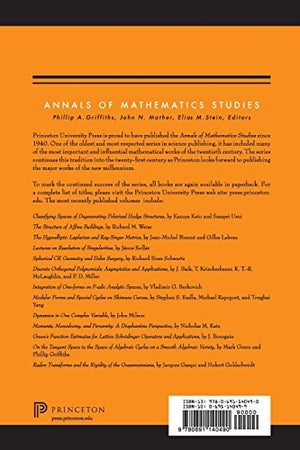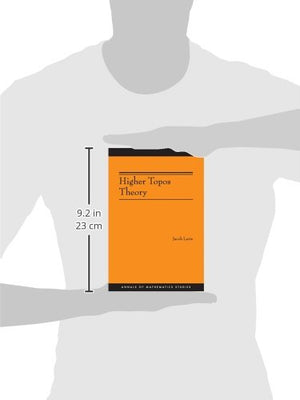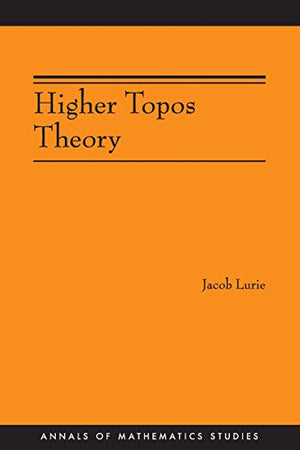# Higher Topos Theory (Am-170) (Annals Of Mathematics Studies)

• Publish Date: 2009-07-26
• Binding: Paperback
• Author: Jacob Lurie
Regular price \$104.27

Attention:For textbook, access codes and supplements are not guaranteed with used items.

Higher category theory is generally regarded as technical and forbidding, but part of it is considerably more tractable: the theory of infinity-categories, higher categories in which all higher morphisms are assumed to be invertible. In Higher Topos Theory, Jacob Lurie presents the foundations of this theory, using the language of weak Kan complexes introduced by Boardman and Vogt, and shows how existing theorems in algebraic topology can be reformulated and generalized in the theory's new language. The result is a powerful theory with applications in many areas of mathematics.

The book's first five chapters give an exposition of the theory of infinity-categories that emphasizes their role as a generalization of ordinary categories. Many of the fundamental ideas from classical category theory are generalized to the infinity-categorical setting, such as limits and colimits, adjoint functors, ind-objects and pro-objects, locally accessible and presentable categories, Grothendieck fibrations, presheaves, and Yoneda's lemma. A sixth chapter presents an infinity-categorical version of the theory of Grothendieck topoi, introducing the notion of an infinity-topos, an infinity-category that resembles the infinity-category of topological spaces in the sense that it satisfies certain axioms that codify some of the basic principles of algebraic topology. A seventh and final chapter presents applications that illustrate connections between the theory of higher topoi and ideas from classical topology.